Reach Us+44-1522-440391
Broadband Dispersion Compensation of Single Mode Fiber by using Modified Decagonal Photonic Crystal Fiber having High Birefringence | OMICS International
Journal of Lasers, Optics & Photonics
All submissions of the EM system will be redirected to Online Manuscript Submission System. Authors are requested to submit articles directly to Online Manuscript Submission System of respective journal.

# Broadband Dispersion Compensation of Single Mode Fiber by using Modified Decagonal Photonic Crystal Fiber having High Birefringence

Aminul Islam Md*

Department of Electrical and Electronic Engineering, Rajshahi University of Engineering and Technology, Rajshahi-6204, Bangladesh

*Corresponding Author:
Aminul Islam Md
Department of Electrical and Electronic Engineering
Rajshahi University of Engineering and Technology
Rajshahi-6204, Bangladesh
Tel: +8801917 028103
E-mail: [email protected]

Received Date: September 12, 2015; Accepted Date: October 17, 2015; Published Date: October 20, 2015

Citation: Islam A (2015) Broadband Dispersion Compensation of Single Mode Fiber by using Modified Decagonal Photonic Crystal Fiber having High Birefringence. J Laser Opt Photonics 2:123. doi:10.4172/2469-410X.1000123

Copyright: © 2015 Islam A. This is an open-access article distributed under the terms of the Creative Commons Attribution License, which permits unrestricted use, distribution, and reproduction in any medium, provided the original author and source are credited.

Visit for more related articles at Journal of Lasers, Optics & Photonics

#### Abstract

This paper presents a highly birefringent dispersion compensating microstructure modified decagonal photonic crystal fiber for broadband transmission communication system. According to simulation, negative dispersion coefficient of -610 ps/(nm.km) and a relative dispersion slope (RDS) close to that of single mode fiber of about 0.0036 nm-1 with birefringence of the order 2.1×10-2 was obtained at 1550 nm. The variation of structural parameters is also studied to evaluate the tolerance of the fabrication. Moreover, effective area, residual dispersion, effective dispersion, confinement loss, and nonlinear coefficient of the proposed modified decagonal photonic crystal fiber (M-DPCF) are also mentioned and discussed.

#### Keywords

Birefringence; Effective mode area; Full-vector Finite Element Method; Photonic crystal fiber; Dispersion compensating fiber; Residual dispersion

#### Introduction

Photonic crystal fibers (PCFs) guide the electromagnetic field by an arrangement of air holes that run down the entire fiber length. In the holey fibers, the air holes reduce the average index around the solid core, and the guidance can be ascribed to the total internal reflection [1,2]. Recently, the most common cladding of PCFs has been investigated extensively [3-6], which consists of circular air holes, arranged in a triangular lattice with symmetric structure. Such holey claddings in PCFs help tuning dispersion slope and controlling confinement losses in a way that was not possible in conventional fibers . PCFs offer flexibility in tuning dispersion [8,9] which is crucial in designing dispersion compensating fiber design. Nowadays, Photonic crystal fibers (PCFs) have diverse applications in telecommunications, sensors, inter-ferometry, soliton, lasers, medical instrumentations and various polarization sensitive devices etc. [10-12]. It is required to compensate the dispersion in the long distance optical data communication system to restrain the broadening of pulse. The way to realize this is to use the dispersion compensating fibers (DCFs) having large negative dispersion . To minimize the insertion loss and reduce the cost, the magnitude of negative dispersion must be as large as possible while the DCFs should be as short as possible. To efficiently compensate the dispersion at all the frequencies of dense wavelength division multiplexing (DWDM), the negative dispersion of DCFs should span a wide spectrum. Again, the dispersion and dispersion slope should be compensated at the same time . Therefore, in designing DCFs, it is important to take into consideration dispersion, dispersion slope, relative dispersion slope, bandwidth, and mode property .

For any fibers with rotational symmetry of an order higher than 2, a mode that has a preferred direction must be one of a pair of degenerate modes . Symmetry in PCFs implies the existence of doublydegenerate pairs of modes that share the same propagation constant (β) and free-space wavelength (λ ), similar to the uniformly polarized HE11 modes in a conventional step-index fiber, so they must be degenerate. When this symmetry in PCFs is affected by some factors, such as bends and twists, stresses, or by manufacturing imperfections, the degeneracy is lifted and the real parts of the effective indices (neff = βλ /2π) of the degenerate modes separate by an amount termed modal birefringence. As a result, the observation of birefringence must be a result of asymmetry in the structure. These perturbations couple the modes that propagate at slightly different phase velocities, with the consequence that the polarization of light becomes unpredictable after a short propagation. Highly Birefringence photonic crystal fibers (HBPCFs) are suitable for various novel applications including sensing application. Here, high birefringence with high negative dispersion coefficient is very urgent. The idea of using PCF for dispersion compensation (DC) was first proposed by Birks et al. .

Several designs have been proposed to obtain a high negative dispersion coefficient or a wide compensation bandwidth for singlematerial microstructure holey fibers (MHFs) . Those MHFs contain air-holes arrayed in a triangular lattice with the same air-hole diameter or with a dual concentric core (with two different air-hole sizes), and they cannot simultaneously have neither a negative dispersion coefficient larger than −600 ps/(nm.km) and a wide compensation bandwidth nor high birefringence. A honeycomb structure MHF with a Ge-doped central core has been proposed for a wide compensation bandwidth and a large dispersion coefficient which can reach −1350 ps/(nm.km)  but the doped core will lead to fabrication difficulties. A MHF with the first ring of special ‘grapefruit’ holes is proposed and its potential as a broadband dispersion compensation fiber is shown . However, the feasibility for practical fiber links has not been numerically studied and the structural variations analyzed are virtually not random in nature, therefore the model does not accurately represent influences from fabrication. In a recent report, an octagonal MHF with the first ring of elliptical holes is proposed which exhibits negative dispersion coefficient of only ‒239.5 ps/(nm.km)  that requires a long length fiber to compensate for the accumulated dispersion of the transmission fiber.

This paper proposes a modified decagonal photonic crystal fiber having high birefringence that may be useful in compensation of dispersion of SMF over a wide range of wavelengths as well as in the field of birefringence application. Through optimizing the two air-hole diameters and scaling the cross-section, the desired dispersion characteristics are efficiently achieved. The effectiveness of the proposed MHF for broadband dispersion compensation for a typical transmission fiber is evaluated, and then the influence of the random imperfections of air-hole 4 diameters is also studied. A full-vectorial finite element method (FEM) has been used to characterize the MDPCF design.

#### Geometries of the Proposed M-DPCF

The proposed M-DPCF is made of pure silica and has a fiber triangular array of air-holes running along its length. The transverse cross-section of the MHF is shown in Figure 1, where Λ is the pitch of the lattice, d1 is the air-hole diameter of the elliptical shaped first ring, a and b are the length of major axis and minor axis respectively of that elliptical shaped first ring, d3 is the air-hole diameter of the third ring and d2 = d4 = d5 = d is the air-hole diameter of the other rings. The airholes of the first ring are relatively large, since it is known that an increase in the air-hole diameter of the first ring causes the dispersion coefficient to decrease for MHFs with a triangular lattice cladding structure .

The air-holes of the third ring are relatively smaller, because a ring of reduced diameter induces a change in the slope of the evolution of the effective index versus wavelength . The influence of any other single outer ring is much more limited ; therefore it is set those rings to have the same air-hole diameter to reduce fabrication complexity. In Figure 1 the background material is pure silica which is enclosed with air-holes .

#### Numerical Method

The finite element method (FEM) with circular perfectly matched boundary layers (PML) is used to simulate properties of the proposed PCF. To model the leakage, an efficient boundary condition has to be used, which produces no reflection at the boundary. PMLs are the inner core outer core most efficient absorption boundary conditions for this purpose . Using the FEM, the PCF cross-section is divided into homogeneous subspaces where Maxwell’s equations are solved by accounting for the adjacent subspaces. These subspaces are triangles that allow a good approximation of the circular structures. Using the PML, from Maxwell’s curl equations the following vectorial equation is obtained .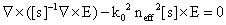(1)

Where E is the electric field vector, k0 is the wave number in the vacuum, neff is the refractive index of the domain, [s] is the PML matrix, [s]-1 is the inverse matrix of [s]. The effective refractive index of the base mode is given as neffβ = k0, where β is the propagation constant. Once the modal effective refractive index neff is obtained by solving an eigen value problem using FEM, the chromatic dispersion D(λ ), confinement loss Lc, and effective area Aeff can be calculated given by . The chromatic dispersion D(λ) of PCFs is calculated using the following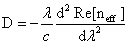(2)

In ps/(nm.km), where Re[neff] is the real part of effective refractive index neff, λ is the wavelength in vacuum, c is the velocity of light in vacuum. The material dispersion can be obtained from the three-term Sellmeier’s formula and it is directly included in the calculation. In PCFs, the chromatic dispersion D (λ) is related to the additional design parameters like geometry of the air-holes, pitch, and whole diameters. By optimizing these parameters, suitable guiding properties can be obtained. Because of the positive dispersion and dispersion slope of the SMF, the fundamental requirements of a DCF for WDM operation are a large negative dispersion and a dispersion slope over a broad range of wavelengths. Assuming that a fiber link consists of a transmission fiber SMF of length LSMF with the dispersion DSMF (λ) and a DCF of length LDCF with the dispersion DDCF(λ), the effective dispersion after compensation, De (λ), on the fiber link in series can be written as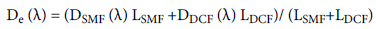(3)

To compensate for the accumulated dispersion of the SMF over a range of wavelengths, the following conditions must be satisfied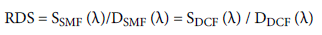(4)

SSMF (λ) and SDCF (λ) are the dispersion slopes for the SMF and DCF, respectively. The unit for the relative dispersion slope RDS is nm-1. Once the RDS of the DCF is close to that of the single mode fiber, SMF, the design of the broadband DCF is accomplished. The RDS is used to judge DC satisfaction over a range of wavelengths. Confinement loss is the light confinement ability within the core region. The increase of air-hole rings help the confinement of light in the core region, which results in lower losses than those with less airhole rings. The confinement loss Lc is obtained from the imaginary part of neff as follows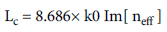(5)

With the unit dB/m, where Im[ neff ] is the imaginary part of the refractive index. The complex refractive index of fundamental mode can be solved from Maxwell’s equations as an eigenvalue problem with the FEM. The effective area Aeff is calculated as follows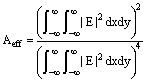(6)

In (μm2), where E is the electric field amplitude in the medium. In PCFs, the birefringence properties are imperative for polarization maintaining applications. PCFs with polarization maintaining (PM) properties are essential in applications such as in eliminating the effect of

Polarization mode dispersion (PMD) and in stabilizing the operation of optical devices, and can also be used in sensing applications. The birefringence is defined as .

B = |nx‒ny| (7)

Where, nx and ny are the mode indices of the two orthogonal polarization fundamental modes. As the holey cladding in PCFs makes the large difference of refractive index between the silica core and cladding, light concentrates more into a very small area of the core, resulting in enhanced effective nonlinearity. A small effective area provides the high optical power density necessary for nonlinear effects to be significant, and the nonlinear coefficient γ calculated by using the following equation .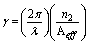(8)

In w-1 km-1, where, n2 is the nonlinear refractive index.

#### Numerical Results

Due to the geometry of the proposed M-DPCF shown in Figure 1, the fiber supports two super modes. One is fundamental superdome, and the other is second order super mode. Guidance occurs in the inner and outer core. The propagation behavior of the PCF is the same as in . At wavelengths shorter than the phase-matching wavelength λ0, the propagation field is essentially confined to the inner core. At wavelengths longer than λ0 the mode field is essentially confined to the outer core. Around λ0, optical coupling between the inner and outer core modes occurs. In the previously reported designs, the inner cladding usually had regular circular air-holes. This gave the inner core strong guidance ability. At wavelengths shorter than the phasematching wavelength, the mode field is trapped tightly in the inner core. Therefore, the dispersion of the PCF is the waveguide dispersion of the inner core mode, which is usually small. Negative dispersion occurs only in a narrow wavelength range around the phase-matching wavelength when the mode field can redistribute from the inner core to the outer core. Such a fiber has only narrowband negative dispersion . To broaden the bandwidth of the negative dispersion first ring of the proposed design is given elliptical shape in which two circular air holes are missing from both side of the ring. By extending the air space of the inner cladding toward the inner core, the inner core is compressed.

The diameter of inner core is higher, which is at the level of the wavelength dimension. The guidance ability of the inner core is weakened. Thus, the mode field cannot be trapped tightly in the inner core, and part of the field leaks out and is coupled to the outer core in a wide wavelength range. It can be considered that part of the fundamental mode field exists in the inner core and part in the outer core. Therefore, the redistribution of the mode field occurs constantly when wavelength varies. This is unlike to the previously reported designs, where redistribution of the field occurs only in the narrow range of wavelength around the phase-matching wavelength. Therefore, the key cause of broadening of the bandwidth of negative dispersion in our proposed design is that elliptical with two missing air-holes in the first ring weaken the inner core’s guidance ability so that field distribution of the fundamental mode occurs whenever the wavelength varies. This redistribution is not limited to a narrow band of wavelength around the phase-matching wavelength.

The dispersion properties of the proposed MHF with different structural parameters have been investigated. As shown in Figure 1, there are three degrees of freedom (d1, d3, and Λ) in the design procedure; those three parameters are adjusted separately and their influence on the dispersion curve is investigated. The dispersion coefficient and dispersion slope at 1550 nm of the SMF is for reference 17 ps/(nm.km) and 0.06 ps nm-2 km-1 respectively, and the calculated RDS is 0.0036 nm-1 .

Figures 2 and 3 show wavelength response of chromatic dispersion and birefringence respectively of the proposed M-DPCF for optimum design parameters. From Figure 2 it is seen that optimizing the parameters Λ, d1, and d3, negative dispersion coefficient of ‒613 ps/ (nm.km) is obtained at 1550 nm. The positions of the air-holes are evident in this figure and it is seen that the mode field is well confined in the core. On the other hand, Figure 3 shows that birefringence of order 2.06×10-2 is obtained at 1550 nm for optimum parameters from the proposed structure.

Figure 2: Wavelength response of chromatic dispersion of the proposed M-DPCF for the optimum design parameters

Figure 3: Wavelength response of birefringence of the proposed MDPCF for the optimum design parameters

After obtaining the optimum dispersion in the way just described in Figure 2, we then have checked the dispersion accuracy of the design. It is known that in a standard fiber draw, ±1% variations in fiber global diameter may occur during the fabrication process. Therefore, roughly an accuracy of ±2% may require ensuring dispersion tolerance . The parameter scan goes from the inner rings to the outer rings. Figure 4 shows the effect of the air-hole diameter of the first ring (d1) on the dispersion behavior with Λ = 0.88 μm, a/Λ = 1.38, b/Λ =0.87, d3/Λ = 0.46, and d/Λ = 0.60. To account for this structural variation, air-hole diameter, d1 is varied up to ±2% from their optimum values. Corresponding dispersion curve is shown in Figure 4 as well as birefringence curve is shown on Figure 5. Solid lines indicate dispersion curves and birefringence curves due to increment in parameters and dashed lines for decrement. It is observed from the Figure 4 and numerical results that the value of dispersion increases smoothly with the increase of first ring air holes diameter while dispersion slope decreases abruptly and vice versa, while Figure 5 show that birefringence decreases with the increase of air hole diameter and vice versa.

Figures 6 and 7 show the effect of the air-hole diameter of the third ring (d3) on the dispersion behavior and birefringence respectively with Λ = 0.88 μm, a/Λ = 1.38, b/Λ =0.87, d1/Λ = 0.67, and d/Λ = 0.60. Here, the Figure 6 and numerical results show that the value of dispersion decreases with the rise of third ring air holes diameter while dispersion slope increases abruptly and vice versa. Figure 7 revels that higher value of air holes diameter increases the value of birefringence and for lower value birefringence decreases gradually.

All of the above procedures have a fixed pitch as Λ = 0.88 μm, and then we scale the cross-section. Figures 8 and 9 show dispersion accuracy and birefringence accuracy respectively of the proposed fiber for pitch, Λ along with the optimum dispersion curve. The pitch varies from about 0.86 μm to about 0.90 μm with normalized air-hole diameters being fixed as a/Λ = 1.38, b/Λ =0.87, d1/Λ = 0.67, d3/Λ = 0.46 and d/Λ = 0.60.

Figure 8: Dispersion properties of MHF: optimum dispersion and effects of changing pitch Λ

Figure 9: Dispersion properties of MHF: optimum birefringence and effects of changing pitch Λ

The results are shown in Figures 8 and 9. The dispersion coefficient is −613 ps/(nm.km) for the optimum design parameters and birefringence is affected slightly for this pitch variation.

Figures 10 and 11 show the effect of the major axis (a) on the dispersion behavior and birefringence respectively with Λ =0.88 μm b/ Λ =0.87, d1/Λ = 0.67, d3/Λ = 0.46 and d/Λ = 0.60 and the effect of the minor axis (b) on the dispersion behavior and birefringence respectively with Λ =0.88 μm, a/Λ = 1.38, d1/Λ = 0.67, d3/Λ = 0.46 and d/Λ = 0.60 are seen in Figures 12 and 13 respectively.

Figure 10: Dispersion properties of MHF: optimum dispersion and effects of changing a

Figure 11: Dispersion properties of MHF: optimum birefringence and effects of changing a

Figure 12: Dispersion properties of MHF: optimum dispersion and effects of changing b

Figure 13: Dispersion properties of MHF: optimum birefringence and effects of changing b

However, the dispersion coefficient and RDS of the reference SMF are respectively 17 ps/(nm.km) and 0.0036 nm-1, the dispersion compensation ratio from 1350 to 1600 nm is shown in Figure 14.

Figure 14: The dispersion compensation ratio (DCR) versus wavelength for the optimum design parameters

The calculation shows that the proposed MHF can compensate a dispersion of the SMF of about 40 times its length, and it can do this to within 10% deviation of the dispersion compensation ratio (DCR) over the entire (1460-1640 nm) 180 nm band centered at 1.55 μm.

Figure 15 shows the wavelength dependence of RDS value for the optimum design parameters:

Figure 15: Dispersion and RDS of the proposed MHF for the optimum design parameters

Λ =0.88 μm, a/Λ = 1.38, b/Λ = 0.87, d1/Λ = 0.67, d3/Λ = 0.46 and d/ Λ = 0.60. The dispersion coefficient and RDS value is – 613 ps/ (nm.km) and 0.0036 nm-1 respectively at 1.55 μm. The RDS value obtained perfectly matches to the single mode fiber RDS value. Figure 16 corresponds to the residual dispersion obtained after the dispersion compensation by 1.135 km long MHF for the dispersion accumulated in one span (40 km) of SMF. It can be observed that the residual dispersion is ±56 ps/nm, which is within the range of ±64 ps/nm over the entire (1460-1640 nm) 180 nm band centered at 1.55 μm, enabling the proposed MHF structure to be suitable for 40 Gb/s transmission system .

Figures 17 and 18 show the confinement loss and effective area for the optimum design parameters. The effective area of the proposed DC-MHFs for the optimum design parameter is 2.8 μm2. The confinement loss remains below 10P-1P dB/m at 1550 nm wavelength for choosing five air-hole rings which is the acceptable level for the transmission fiber. It is already shown that the proposed MHF can exhibit negative dispersion, better compensation ratio, and low confinement losses.

Figure 17: Wavelength dependence of effective area of the proposed MHF for the optimum design parameters

Figure 18: Wavelength dependence of confinement loss of the proposed MHF for the optimum design parameters

#### Conclusion

In this letter, a simple MHF structure is proposed to achieve a high negative dispersion coefficient of ‒613 ps/(nm.km) with birefringence of the order 2.1×10-2, while retaining perfect matching of the relative dispersion slope to a standard single mode fiber at 1.55 μm. Through the optimization of geometrical parameters, a broadband dispersion compensating MHF can be efficiently designed. Furthermore it is shown that allowing 10% deviation of the dispersion compensation ratio, broadband dispersion compensation of SMF over a 180 nm wavelength range can be obtained by using the proposed dispersion compensating fiber design. Finally, its tolerance for fabrication imperfections is also analyzed, and the results show that this structure is robust enough for practical applications. This fiber has a modest number of design parameters, five rings, three air-hole diameters, and an air-hole pitch.

#### References

Select your language of interest to view the total content in your interested language

### Article Usage

• Total views: 9252
• [From(publication date):
December-2015 - Aug 17, 2019]
• Breakdown by view type
• HTML page views : 9057
• PDF downloads : 195

## Post your commentCan't read the image? click here to refresh
###### Peer Reviewed Journals

Make the best use of Scientific Research and information from our 700 + peer reviewed, Open Access Journals

International Conferences 2019-20

Meet Inspiring Speakers and Experts at our 3000+ Global Annual Meetings

Top